# Double contour integral.

evol_w10lv

## Homework Statement

I have got double contour integral: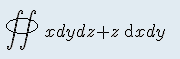And here is picture: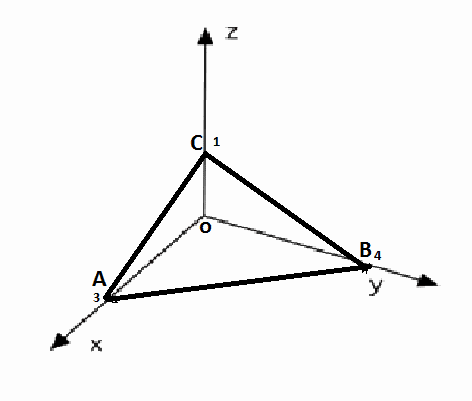I know every line equation as well.
The task is to calculate this integral as:
1. surface integral of outside of the area (in the picture)

## Homework Equations

Equation of plane: 4x+3y+12z-12=0
It means that x=3-0.75y-3z and z=1-0.25y-(1/3)x

## The Attempt at a Solution

I guess, this can be integral for task 1, when we use surface integral: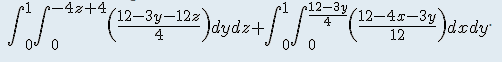Can someone chek, if it's correct? And how to start task 2, when I have to use Ostrogradsky formula?

jackmell

## Homework Statement

I have got double contour integral:And here is picture: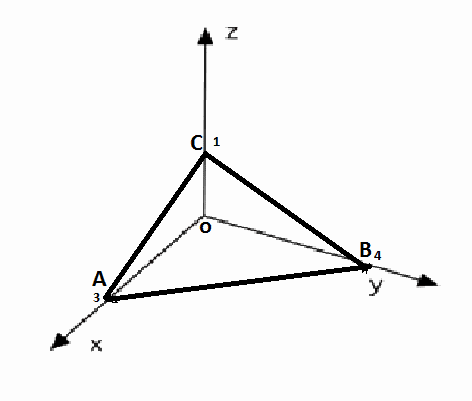I know every line equation as well.
The task is to calculate this integral as:
1. surface integral of outside of the area (in the picture)

## Homework Equations

Equation of plane: 4x+3y+12z-12=0
It means that x=3-0.75y-3z and z=1-0.25y-(1/3)x

## The Attempt at a Solution

I guess, this can be integral for task 1, when we use surface integral: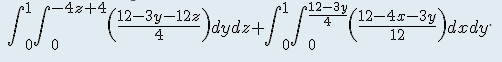Can someone chek, if it's correct? And how to start task 2, when I have to use Ostrogradsky formula?

What are we going to do with this evol? I have some questions and observations:

(1) Isn't the (real) double contour integral above just a surface integral?

(2) If this is a surface integral, then the double integrals you're written are not correct. The integrals you wrote are just simple volume integrals. Also, the outer limits of the second integral are incorrect for a volume integral: the upper limit on y is 4 and not 1.

(3) Your statement above, "1. surface integral of outside of the area (in the picture)" is not clear at all. What area? Do you mean the surface integral over the triangular region intersecting the axis planes? Would be better to say something like, compute the surface integral:

$$\mathop\iint\limits_{\text{Red}} x dS$$

Where "red" is the surface in the plot below. What would that surface integral be?

#### Attachments

Last edited:
evol_w10lv
I guess, it's just surfice integral.
It means that this is the correct solution: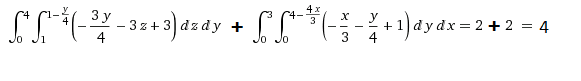?

jackmell
I guess, it's just surfice integral.
It means that this is the correct solution: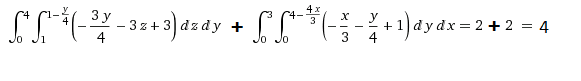?

I don't think so. Remember the formula for a surface integral over the surface z=f(x,y)? It's

$$\mathop\iint\limits_S g(x,y,z)dS=\mathop\iint\limits_R g(x,y,f(x,y)) \sqrt{f_x^2+f_y^2+1}dA$$

evol_w10lv
Hmm.. I tried to use this example: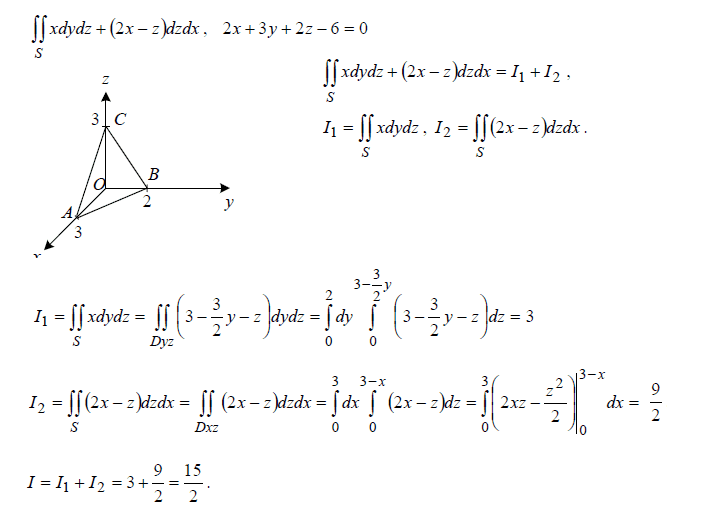Why in that case my solution is wrong?

jackmell
Hmm.. I tried to use this example:Why in that case my solution is wrong?

Hi evol,

I don't wish to steer you wrong. Perhaps I'm misunderstanding what you're trying to do here. Those integrals above are just volume integrals and not what I believe are surface integrals. Maybe though I'm not understanding this problem.

Last edited:
evol_w10lv
I guess, I don't understand this problem as well.
I will try to find out, what exactly I have to calculate.